- JoNova - http://joannenova.com.au -

New Science 3: The Conventional Basic Climate Model — In Full

Posted By David Evans On September 25, 2015 @ 1:57 am In Global Warming | Comments Disabled

A feast. A feast!  For those who want the meat, the math and the diagrams (don’t miss the diagrams). As far as we know, this is the first time the architecture of the basic climate model has been laid out in one place on the Net. This is the most math heavy post this series, but it has to be done, and properly. This is where the 1.2 °C direct effect of doubling CO2 gets amplified to 2.5 °C with fairly basic physics. If the equations are not your forte, look at the “the Establishment Case” below the equations to get some idea why establishment scientists find it mind-bendingly hard to imagine how climate sensitivity could possibly be much different.

For commenters who know there are problems with this model (don’t we all), one of the points of doing this is to get through to the establishment leaders — to speak their language instead of having separate conversations. Of course, for some minds it will not matter what skeptical scientists say, but for other, key people, it will. We would expect seeing the flaws laid out so clearly will undercut the implacable confidence of leaders, though they may not say so.

Again, this ties for Most Uncontroversial Post on this blog. Everything here, the IPCC would agree with (except maybe the last sentence).

Do admire the diagram –Figure 2. It’s no accident it is similar to an electrical circuit diagram. Modeling and feedbacks have been tested to the nth by thousands of electrical engineers around the world building things we use every day. Thinking of the climate model this way is a useful technique to figure out where it goes wrong.

In a sense this is where silicon chip wisdom starts to scrutinize the climate maze.

– Jo

# 3. The Conventional Basic Climate Model — In Full

Dr David Evans, 24 September 2015, David Evans’ Basic Climate Models Home, Intro, Previous, Next, Nomenclature.

Here is the conventional basic climate model, in full. It builds on the previous post in the series, which explained how the model worked in the case of no feedbacks and only a CO2 input. This post uses the same terminology, notation, and ideas, without necessarily explaining them again. This is “heaviest” post in the series.

Readers who don’t want to see details of the mathematical modeling might want to skip straight to the diagram and the calculation of ECS at the end — a general understanding of the previous post and of the model diagram below is sufficient to make sense of the ensuing posts in this series.

### The Set-Up: Multiple Influences and Multiple Feedbacks

Consider the hypothetical situation where only the only things that can change are:

• Temperature. While some variables might alter the relativities between various mean atmospheric temperatures, we will take the temperature to be the one we are most interested in, the surface temperature TS.
• The n driver variables, V1,…,Vn, are the climate influences considered in the model, such as the CO2 concentration, the total solar irradiance (TSI), or the externally-driven albedo (EDA). The driver variables are not directly dependent on the temperature, each other, or the feedback variables.
• The m feedback variables, U1,…,Um, are the feedbacks that the model considers, such as the average lapse rate, the surface-driven albedo, or the average height of the water vapor emissions layer (WVEL). Each feedback variable is dependent on the temperature, but is not directly dependent on the driver variables or on the other feedback variables. Because they depend on temperature, as the Earth warms these variables further affect absorbed solar radiation (ASR) or outgoing longwave radiation (OLR), which affects warming, which further affects these feedback variables, and so on in a feedback loop.

The previous post is the special case where n equals one, m is zero, and V1 is the base-2 logarithm of the CO2 concentration L.

We are going to focus on the balance of radiation entering and leaving the planet, so it is helpful to explicitly focus on the net top-of-atmosphere (TOA) downward flux, namely### How Much Does the OLR Change?

If the temperature, the drivers, and the feedbacks all change, how much does the OLR change?

We generalize the process that led to Eq. (3) of the previous post. G depends on TS as well as on each of the driver and feedback variables, but on nothing else because everything else is held constant. The arguments of G are treated as 1+n+m independent variables, each of which varies by an incremental amount (using the “d” notation). We apply the well-known calculus rule for partial derivatives: the total change in G is the sum of the changes due to each variable, and the change due to a variable is the product of the partial derivative of G in that variable with the actual change in that variable –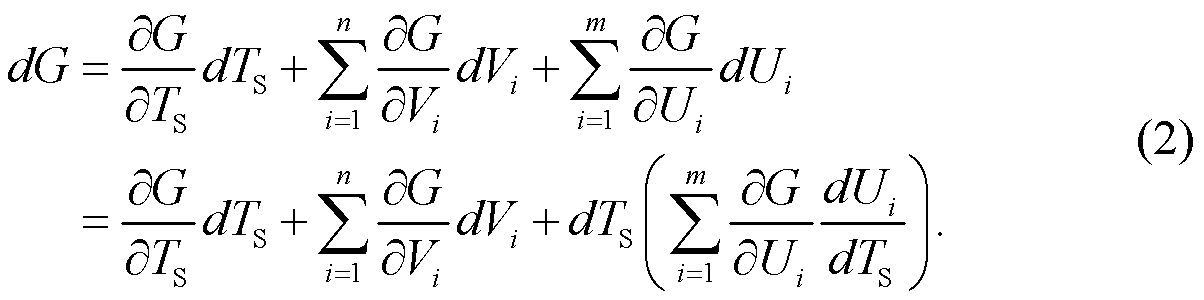(The Σ symbols means to sum what follows, one term for each value of the index i, whose values are in turn 1, 2,…, n or m. Thus each Σ is the sum of n or m terms, where each of the n or m terms in that sum is like what follows the Σ but with the appropriate value substituted for i.)

### The Balance between Temperature and the Climate Influences

As in the simple case, we convert this description of how OLR changes into a description of a balance between increases and decreases in net flux, by considering a move between two steady states. Assuming the planet stays close to steady state as the climate influences do their work, the incremental changes dX become small changes ΔX, so Eq. (2) becomes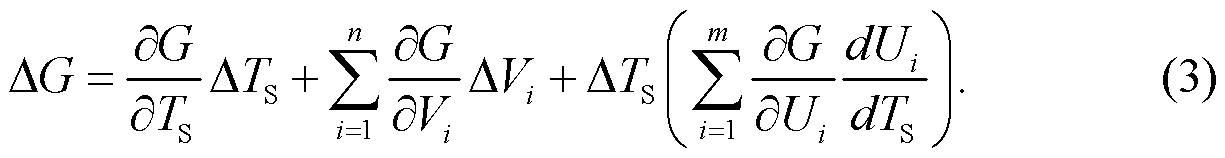The radiation is balanced in a steady state (i.e. G is zero), so there is no change in net downward flux between steady states, so ΔG is zero. Also, because ASR is not directly dependent on surface warming, by Eq. (4) of the previous post,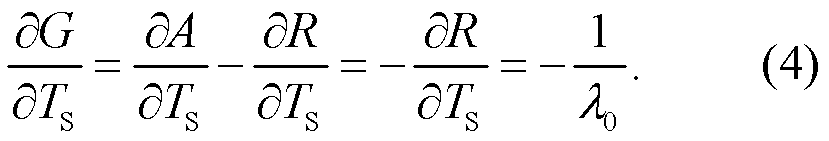Applying these two relationships to Eq. (3) gives the equation describing the balance: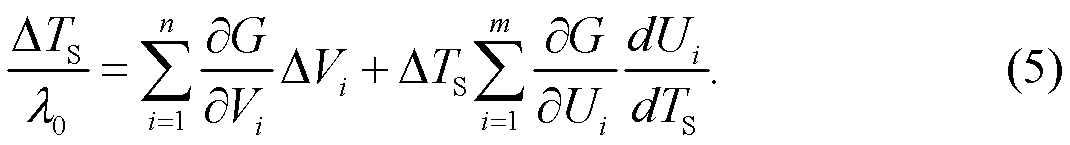Ignoring the feedbacks  (by pretending all the Ui and their derivatives are zero), this says that the increase in OLR due to surface warming is balanced by an increase in net downward flux (i.e. increases in ASR plus decreases in OLR) due to changes in the driver variables V1,…,Vn.

### Interpretation

Changes in the drivers  can be viewed as a process with n+1 stages:

• In stage i, where i is in {1,2,…,n}, the climate driver variable Vi changes by ΔVi and everything else is held constant, which increases the net TOA downward flux G by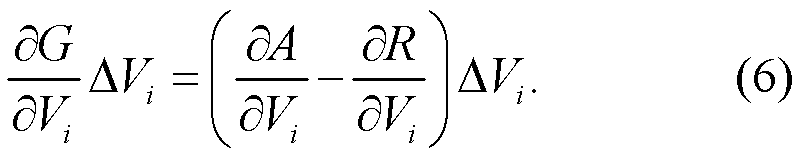• In stage n+1, the surface warms by ΔTS holding all the drivers constant, which increases OLR by exactly enough to restore the net change in G to zero.

Or these n+1 stages can be viewed as n+1 processes that occur simultaneously, without significantly interfering with each other, and we add the OLR changes that each causes (the climate is linear for small changes, so processes and changes superpose).

### Feedbacks

Feedbacks complicate the balance in Eq. (5) by adding yet more downward flux (which can be negative) due to changing values of the feedback variables U1,…,Um, which change in response to surface warming. These extra downward fluxes have to be balanced by yet more OLR due to surface warming, requiring more surface warming, which further changes the values of the feedback variables, and so on in a feedback loop.

Solving Eq. (5) for ΔTS, the surface warming is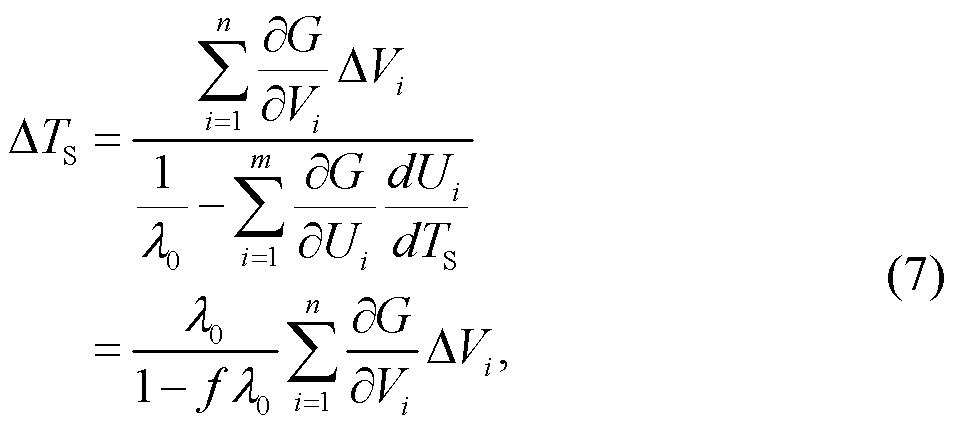where the total feedback f is defined as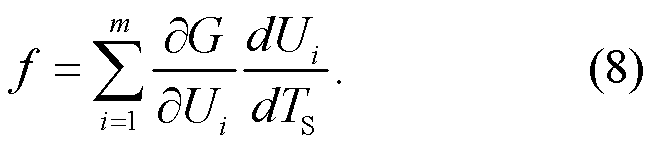This expression for ΔTS is the same as it is without feedbacks (i.e. with f equals zero) except that it is multiplied by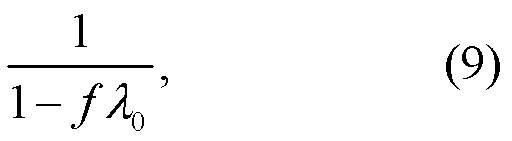which is the distinctive form of a feedback f around a multiplier λ0 — apply Fig. 1 with a equal to λ0 and b equal to f.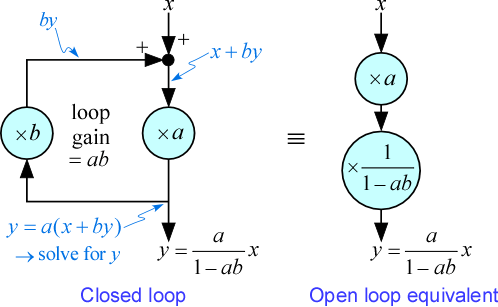Figure 1: The closed-loop and open-loop forms of a feedback loop are equivalent.

AR5 reports the individual and total feedbacks from the CMIP5 as (Table 9.5 and Fig. 9.43, and p. 591), in W m−2 °C−1:

• water vapor +1.6±0.3
• lapse rate −0.6±0.4
• water vapor and lapse rate combined +1.1±0.2
• surface albedo +0.3±0.1,
• cloud +0.3±0.7,

and the total feedback is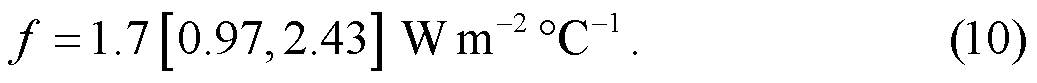(Incidentally, the loop gain, the total amplification going once around the feedback loop, isIt is less than one. If it were greater than or equal to one then the infinite sum implicit in the denominator in Eq.s (7) and (9) would not converge and the computed surface warming would be infinite.)

In Eq. (7) the contribution to the surface warming by every driver shares a part in common: the Planck sensitivity λ0, modified by the feedbacks f. This inspires the concept of the “forcing” of a driver, as the part peculiar to the driver variable: the (instantaneous radiative) forcing of a change ΔVi  in the climate driver variable Vi  is the resulting change to the net TOA downward flux, namely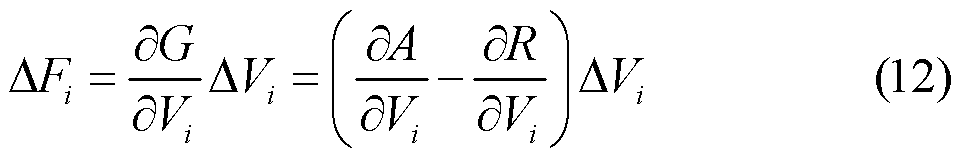(see AR5, section 8.1.1.1 and Fig. 8.1; this is the appropriate form of forcing for changes between steady states). The contribution the change in climate driver Vi makes to surface warming, before feedbacks, is the product of its forcing and the Planck sensitivity: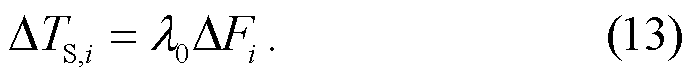The (total) radiation imbalance is the total change in downward flux before feedbacks, or the sum of forcings due to the drivers: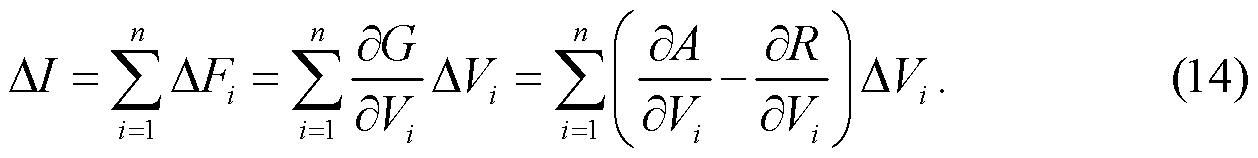### Diagram

The situation is illustrated in Fig. 2:

• The first driver, V1, is the no-feedbacks ASR ANF, the portion of ASR that is unaffected by feedbacks in the period under consideration. (Moving between steady states, changes in ASR are due either to ΔANF or to albedo feedbacks. Changes in ANF are caused by something external to Earth, such as a fluctuation in TSI or a change in externally-driven albedo.)
• The second driver, V2, is the logarithm of the CO2 concentration, L.
• The feedbacks are all combined into the total feedback f, see Eq. (10) above.
• The computational paths from the drivers to the surface warming ΔTS  are provided by Eq. (7), noting that (see Eq. (5) of the previous post for the last)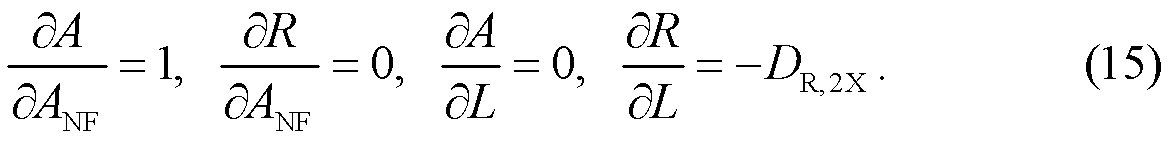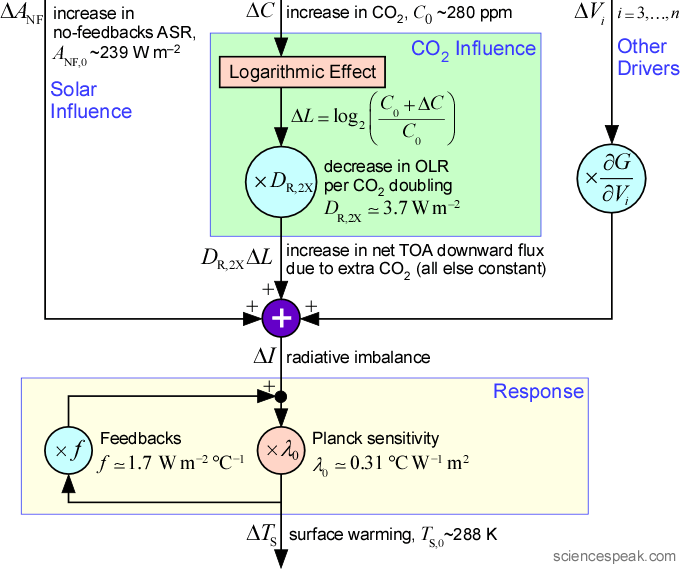Figure 2: Conventional basic climate model, for changes from one steady state to another. The radiation imbalances (or forcings) due to the drivers are added (the purple circle), then balanced by the responding increase in feedbacks-adjusted OLR due to surface warming that would occur if all else was constant.

Adding the forcings and applying the feedbacks in Fig. 2, the surface warming is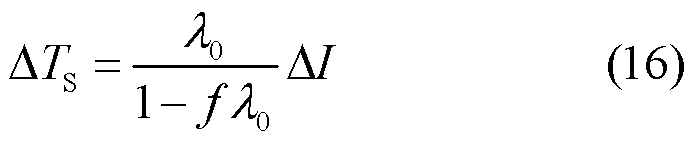where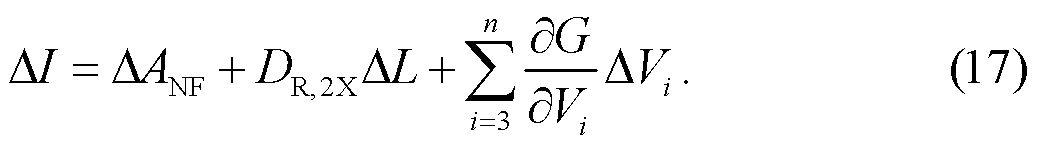Each driver adds its forcing to the input of the Planck sensitivity.

### The Conventional Calculation of the ECS

The equilibrium climate sensitivity (ECS) is the surface warming ΔTS when the CO2 concentration doubles (i.e. ΔL is one) and the other drivers are unchanged, which by Eq.s (16) and (17) is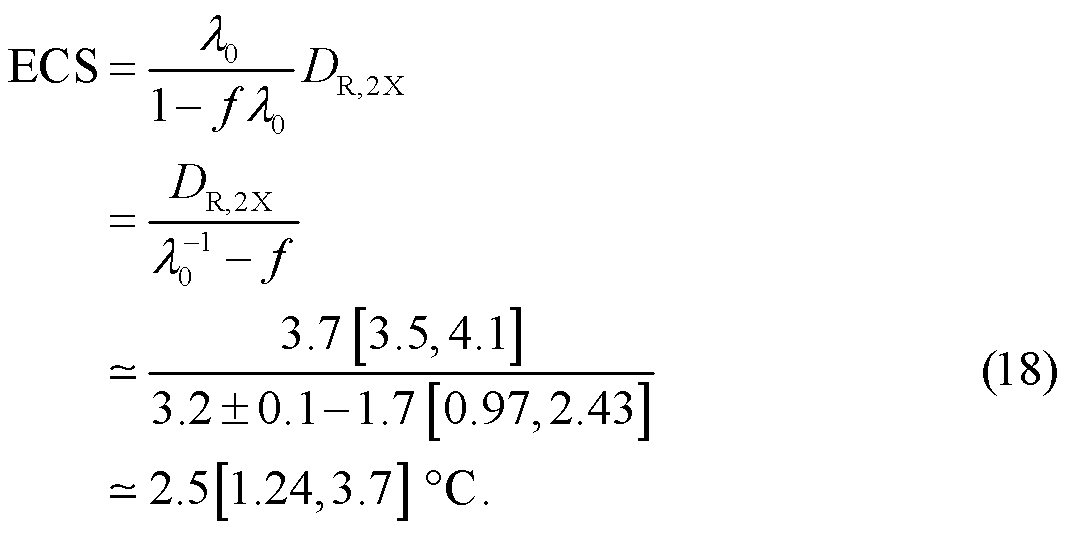This agrees with AR5 (p. 1033), which finds the ECS as likely to be 1.5°C to 4.5°C (for all greenhouse gases, not just for CO2, because it is for a doubling of equivalent CO2 concentration — AR5 p.1451, p.1452). Setting f to zero in Eq. (18) gives the no-feedbacks-ECS found in the previous post.

### The Establishment Case

This calculation in Eq. (18) shows that the sensitivity of carbon dioxide is about 2.5 °C (though in AR4 (2007) the central value was higher, about 3.3 °C), though there is a pretty wide uncertainty. Note that the three parameters in Eq. (18) were obtained mainly by basic physics:

• DR,2X, the decrease in OLR if CO2 doubles holding all else constant, is based mainly on spectroscopy that can be replicated in laboratories.
• λ0, the Planck sensitivity, comes from Stefan-Boltzmann’s law (though it gets modified by 20% or so because we are concerned here with the surface temperature rather than the radiating temperature of the Earth). Stefan-Boltzmann’s law is based on replicated laboratory physics, and is almost certainly beyond reproach.
• f, the total feedback, comes from the large computer models, but its main components are arguably based largely on known physical properties of water vapor and so are surely about right. Although the possibility exists that there is a large and unknown negative feedback that has inadvertently been omitted, it hasn’t been found despite decades of searching.

The first doubling of CO2 from pre-industrial levels level might occur by around 2070, and it is widely thought that the CO2 level will top out at most after about three doublings, in the next century or two. If three doublings from pre-industrial occur, the expected surface warming by Eq. (18) will be 7.5 [4.5,16.5] °C. Even 4.5 °C would be pretty disruptive, while the upper end possibilities would be disastrous. Hence the alarm.